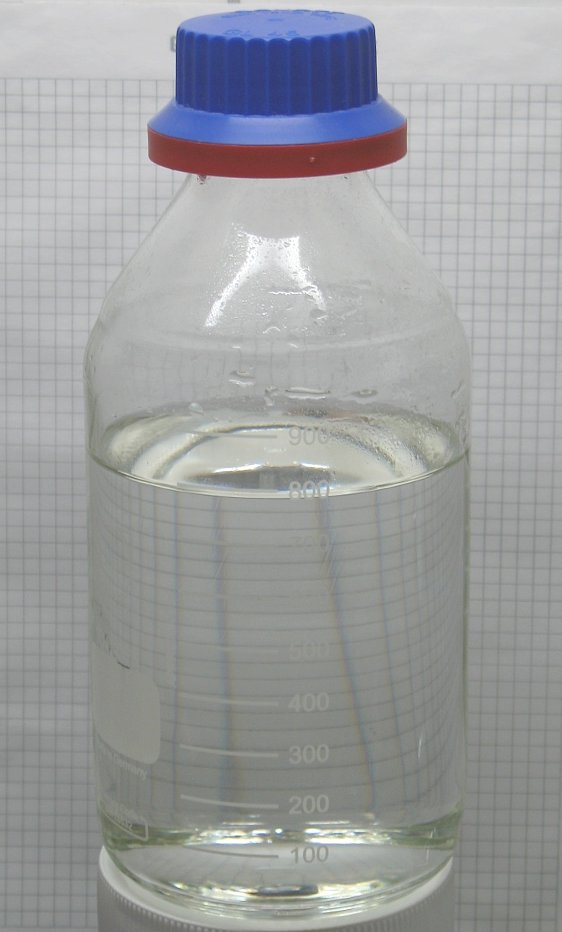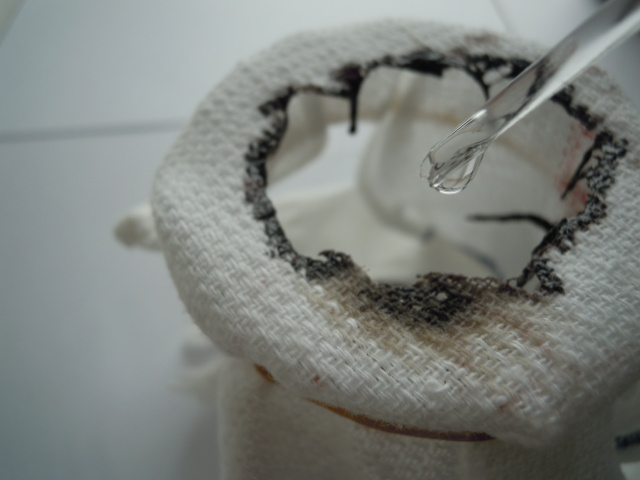## Binary Acids

Binary acids are certain molecular compounds in which hydrogen is bonded with a nonmetal.

### Learning Objectives

Explain the periodic trends that affect binary acid strength.

### Key Takeaways

#### Key Points

• A binary acid ‘s strength depends on the H-X bond ‘s strength; the weaker the bond, the stronger the acid.
• The names of binary acids begin with “hydro-” followed by the name of the other element, modified to end with “-ic.”
• Binary acids are one of two classes of acids; the second are oxoacids (or oxyacids), which consist of hydrogen, oxygen, and another element.

#### Key Terms

• binary acid: molecular compounds in which hydrogen is combined with a second nonmetallic element
• pKa: a quantitative measure of the strength of an acid in solution; a weak acid has a pKa value in the approximate range −2 to 12 in water, and a strong acid has a pKa value of less than about −2.

### Acid Strength and Bond Strength

Binary acids are certain molecular compounds in which hydrogen is combined with a second nonmetallic element; these acids include HF, HCl, HBr, and HI.

HCl, HBr, and HI are all strong acids, whereas HF is a weak acid. The acid strength increases as the experimental pKa values decrease in the following order:

HF (pKa = 3.1) < HCl (pKa = -6.0) < HBr (pKa = -9.0) < HI (pKa = -9.5).Hydrochloric acid: Hydrochloric acid is a clear, colorless solution of hydrogen chloride (HCl) in water. It is a highly corrosive, strong mineral acid with many industrial uses. Hydrochloric acid is found naturally in gastric acid. It is a member of the binary acids.

Why is HF a weak acid, when the rest of the hydrohalic acids are strong? One might correctly assume that fluorine is very electronegative, so the H-F bond is highly polar and we can expect HF to dissociate readily in solution; this reasoning is not wrong, but the electronegativity argument is trumped by considerations of ionic size. Recall the periodic trend that ionic size increases as we move down the periodic table. Because fluorine is at the top of the halogens, the F ion is the smallest halide; therefore, its electrons are concentrated around its nucleus, and as a result, the H-F bond is relatively short. Shorter bonds are more stable, and thus the H-F bond is more difficult to break.

Once we move down to chlorine, howver, the trend changes. Chlorine is larger and has more electrons, and therefore the H-Cl bond is longer and weaker. In the presence of water, the electrostatic attractions between water’s partially negative oxygen and the partially positive hydrogen on H-Cl is strong enough to break the H-Cl bond, and the ions dissociate in solution.

The same reasoning applies for both HBr and HI. These acids are even stronger than HCl because the Br and I ions are even larger. As such, the H-Br and H-I bonds are even weaker, and these compounds also readily dissociate in solution.

### Naming Binary Acids

The names of binary acids begin with “hydro-” followed by the name of the other element, modified to end with “-ic.” For example, HCl is named hydrochloric acid.

Binary acids are one of two classes of acids, the second being oxoacids (or oxyacids), which consist of hydrogen, oxygen, and a third element, which is often a non-metal.

## Oxoacids

An oxoacid is an acid that contains oxygen.

### Learning Objectives

Discuss the periodic trends that help determine oxoacid strength.

### Key Takeaways

#### Key Points

• The central atom ‘s electronegativity and the number of oxygen atoms determines oxoacid acidity.
• Carboxylic acids are an important subclass of organic oxoacids and the most common type of organic acid.
• Carboxlic acids are characterized by the presence of at least one carboxyl group and have a general formula of R-COOH, where R is some monovalent functional group.

#### Key Terms

• oxyacid: an acid containing oxygen, as opposed to a hydracid (oxoacid)
• carboxylic acid: any of a class of organic compounds containing a carboxyl functional group (a carbon with one double bond to an oxygen and a single bond to another oxygen, which is in turn bonded to a hydrogen)

An oxoacid (sometimes called an oxyacid ) is an acid that contains oxygen. To be more specific, an oxoacid is an acid that:

1. contains oxygen
2. contains at least one other element
3. has at least one hydrogen atom bonded to oxygen
4. forms an ion by the loss of one or more protons in solution.

Examples of oxoacids include:

• Carboxylic acids
• Sulfuric acid
• Nitric acid
• Phosphoric acid

Halogen oxoacids include hypochlorous acid (HOCl); chlorous acid(HOClO); chloric acid(HOClO2); oerchloric acid(HOClO3); oerbromic acid (HOBrO3)

All oxoacids have the acidic hydrogen bound to an oxygen atom, so bond strength (length) is not a factor, similar to binary nonmetal acids; instead, the main determining factor for an oxacid’s relative strength has to do with the central atom’s electronegativity (X), as well as the number of O atoms around that central atom.Sulphuric acid: Drops of the concentrated oxoacid sulfuric acid (sulphuric acid) rapidly dehydrate a piece of cotton towel.

### Electronegativity of the Central Atom

Consider the simple oxyacids HOI (hypoiodous acid), HOBr (hypobromous acid), and HOCl (hypochlorous acid). These acids can be arranged in order of their pKavalues and, by extension, their relative strengths:

HOCl pKa = 7.5 < HOBr pKa = 8.6 < HOI pKa = 10.6

Recall that smaller values of pKa correspond to greater acid strength. Therefore, HOCl is the strongest acid and HOI is weakest, and acid strength decreases as the central halogen descends on the periodic table.

The strength of the acid is determined by the central atom’s electronegativity relative to the surround atoms in the molecule. Because Cl is the most electronegative, it draws the bulk of the electrons in the HOCl molecule toward itself; because H and Cl are on opposite ends of the molecule, Cl pulls at the electrons in the H-O bond, thereby weakening it. The weaker the H-O bond, the more easily the H+ can ionize in water, and the stronger the acid.

### Number of Oxygen Atoms Around the Central Atom

Consider the family of chlorooxoacids, which are arranged below in order of pKa values:

HOClO3 pKa = -8 < HOClO2 pKa = -1.0 < HOClO pKa = 1.92 < HOCl pKa = 7.53

The strongest acid is perchloric acid on the left, and the weakest is hypochlorous acid on the far right. Notice that the only difference between these acids is the number of oxygens bonded to chlorine. As the number of oxygens increases, so does the acid strength; again, this has to do with electronegativity. Oxygen is a highly electronegative element, and the more oxygen atoms present, the more that the molecule’s electron density will be pulled off the O-H bond, weakening it and creating a stronger acid.

### Carboxylic Acids

Carboxylic acids are an important subclass of organic oxoacids, characterized by the presence of at least one carboxyl group. The general formula of a carboxylic acid is R-COOH, where R is some monovalent functional group. A carboxyl group (or carboxy) is a functional group consisting of a carbonyl (RR’C=O) and a hydroxyl (R-O-H), which has the formula -C(=O)OH, usually written as -COOH or -CO2H.A carboxylic acid: Carboxylic acids are organic oxoacids characterized by the presence of at least one carboxyl group, which has the formula -C(=O)OH, usually written as -COOH or -CO2H.

Carboxylic acids are the most common type of organic acid. Among the simplest examples are formic acid H-COOH, which occurs in ants, and acetic acid CH3-COOH, which gives vinegar its sour taste. Acids with two or more carboxyl groups are called dicarboxylic, tricarboxylic, etc. The simplest dicarboxylic example is oxalic acid (COOH)2, which is just two connected carboxyls. Mellitic acid is an example of a hexacarboxylic acid. Other important natural examples include citric acid (in lemons) and tartaric acid (in tamarinds).

Salts and esters of carboxylic acids are called carboxylates. When a carboxyl group is deprotonated, its conjugate base, a carboxylate anion, forms. Carboxylate ions are resonance stabilized, and this increased stability makes carboxylic acids more acidic than alcohols. Carboxylic acids can be seen as reduced or alkylated forms of the Lewis acid carbon dioxide; under some circumstances they can be decarboxylated to yield carbon dioxide.

Sulfuric Acid and Sponge Reaction in Slow Motion by Slow Mo Lab: Sulfuric acid dissolves a sponge in slow motion